# Aligning Planning Models with Real-World Observations

## Problem setting

In this work, we aim to improve the predictions of a computer vision model through aligning a sequence of images and their predictions with a provided graph structure of the problem. This problem is highlighted below.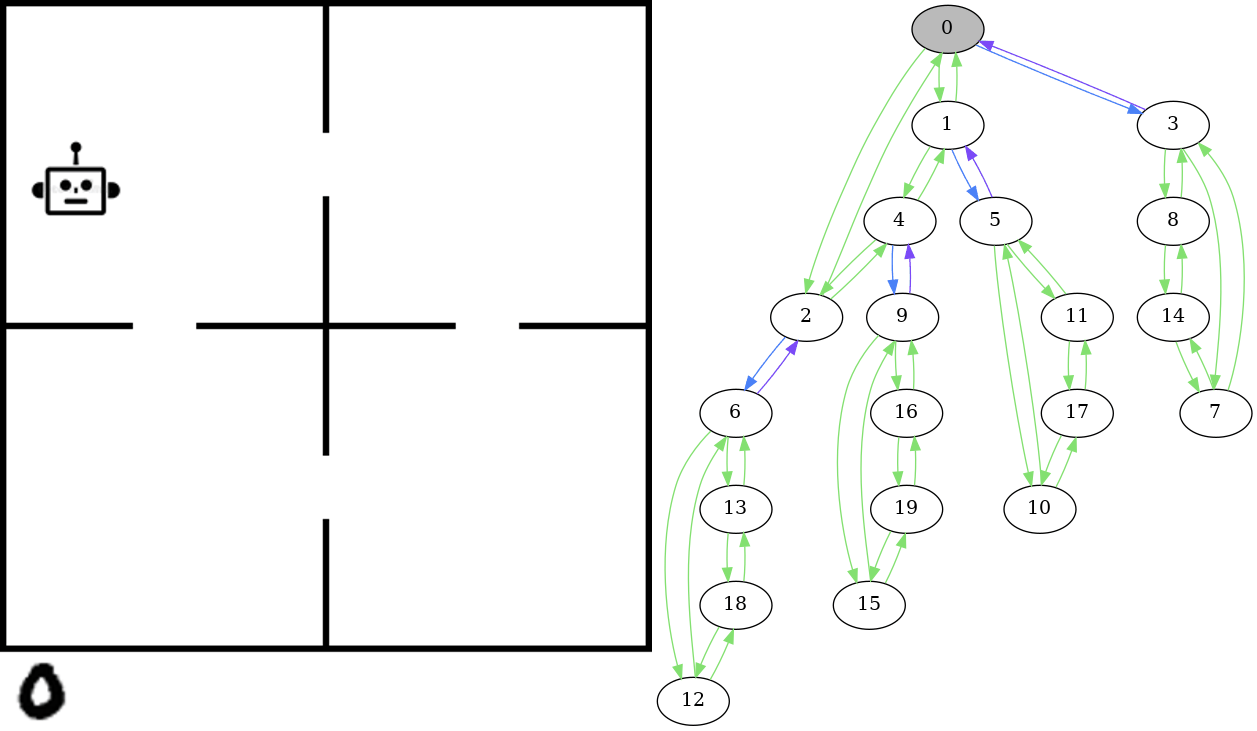On the left is an example of the kind of data we’re looking at: sequences of images showing actions taking place in an environment. These are examples of the images the computer vision model is predicting the underlying state of. In this environment, the actions are the agent picking up objects (represented by the number 0 for this example) and moving between rooms. The robot can pick up the object (represented by the object moving to the bottom left corner outside the rooms) and place it in the robots current room. The idea is that the state should be recognized as being the same no matter where in the room the robot or the object is located - all that matters is the room they are in. Furthermore, the appearance of the object changes between states - we sample this from the MNIST dataset of handwritten numbers to obtain variation.

The graph on the right shows the state space - this is essentially a model of how we know the environment to behave. The state of the image on the left is highlighted in grey. In this graph, there is an edge from one state to another if there is a valid transition between those states, in this case if there is an action in the corresponding planning domain of the problem that transitions from one state to the other. Given the sequence of images such as those shown on the left in the gif, we expect that the corresponding states form a path in the graph. That is, there is a valid transition occuring between each sequential pair of states in the sequence.

## Goal

Given a sequence of images representing states in a given state-space, we want to train a model to predict the underlying state for each image. Futhermore, we want the sequence of predictions to align with a given state-space graph that dictates which transitions are valid in the environment.

## The data

First, we generate some data using planning domains as a testbed.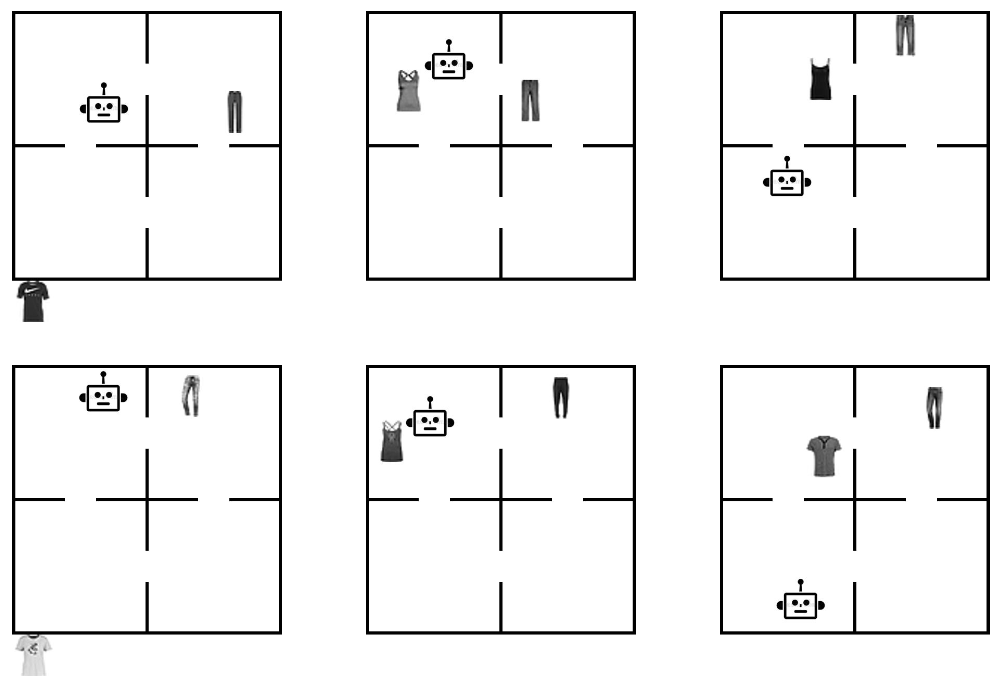Here, both rows show a sequence of the same 3 states. In these examples the objects are sampled from the Fashion MNIST dataset, each object represented as a different item of clothing. Each image on the top is the same state as the one below it, but visually they appear different due to sampling different items of clothing and the position of objects. The challenge here is for the model to learn concepts like “t-shirt” and “pants” to differentiate the objects, as well as understanding which room an object is in when the objects position in the room can change between states.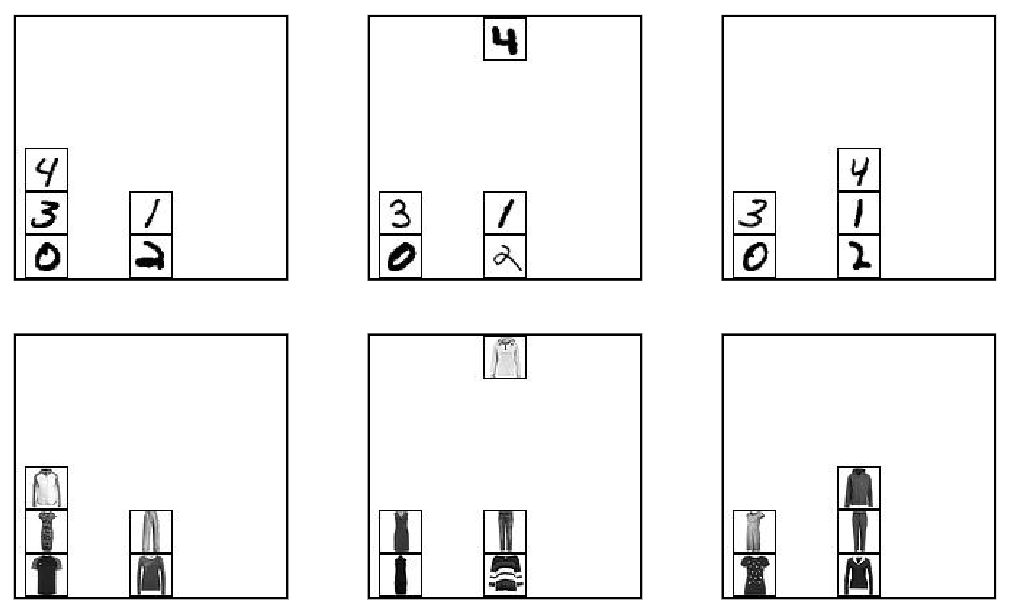Here, labeled blocks are moved around on a table. The model needs to differentiate between the blocks, and understand what state the specific arrangement of blocks corresponds to. Above, the labels are numbers sampled from MNIST. Below, they are represented by different articles of clothing sampled from Fashion MNIST for increased difficulty.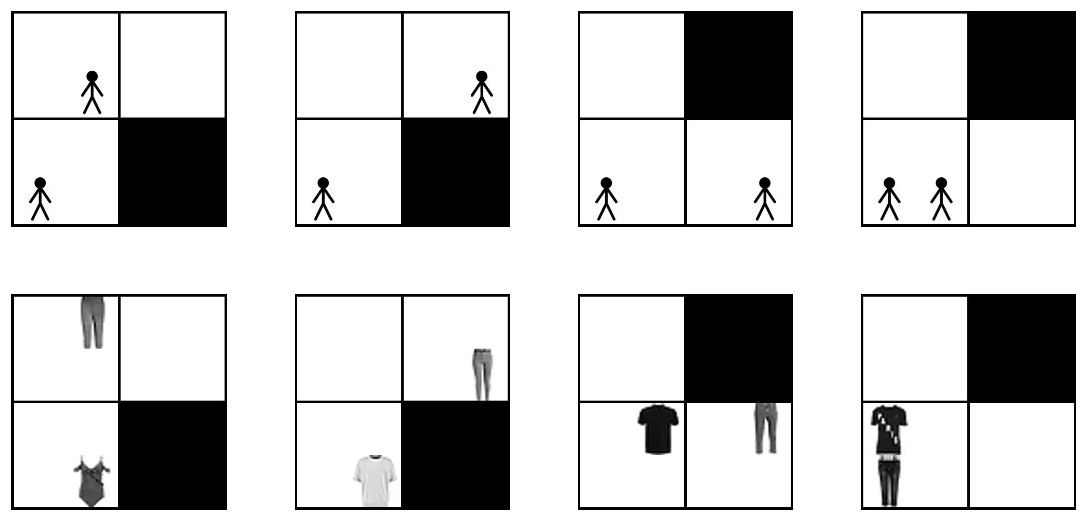This domain models people taking an elevator, where they are shown waiting at different floors on the left half, and the elevator is shown on the right half. Above, we highlight the mechanics using stick figures, showing a person on the 2nd floor getting on the elevator and getting off at the first floor. Below shows what the equlivalent states look like in our training data: representing different people as different clothing items, and assigning them locations within the waiting area or elevator randomly to add difficulty disambiguating between states.

## The model

To predict the state we use contrastive learning. This is a deep learning framework that aims to represent images belonging to the same class similarly, while distancing these representations from those of images belonging to a different class. In our case, examples of images representing the same underlying state should have representations that are similar, yet different and identifiable from representations of different classes. We experimented with several contrastive methods before deciding to use SupCon, of which the general architecture is depicted and described below.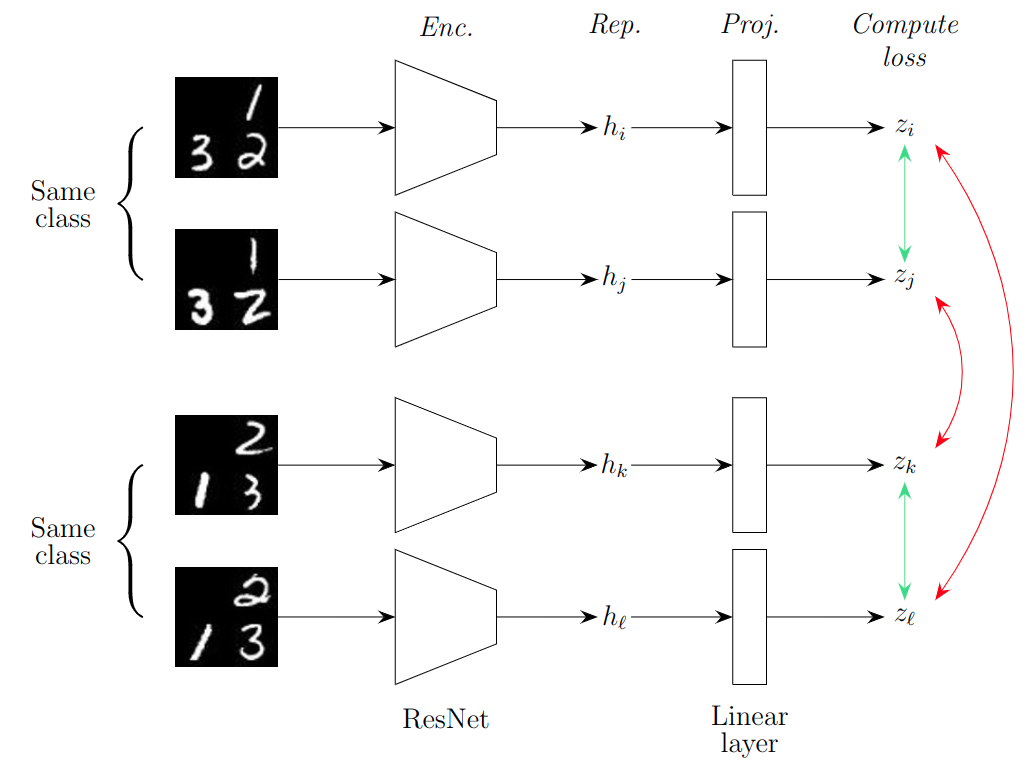The first two images belong to the same class and hence should be represented similarly, as represented by the green arrow on the right which represents pulling the representations together. The bottom pair of states are also both the same, but different from the top two states. Hence, their representations should be different, as represented by the red arrows on the right side of the figure. The network has two parts, an encoder (‘Enc.’) and a projector (‘Proj.’). While the contrastive loss is applied on the output of the projector, the representation used for downstream tasks is the one after the encoder but before the projector, labelled as ‘Rep.’ in the figure.

Not shown in this figure is an additional linear layer after ‘Rep.’ (the $h$’s), this layer has an output neuron for every state in the state-space, acting as a classifier for the final state. We convert the output of this layer (without any activation functions applied) to a probability distribution to obtain the ‘probability’ of the input image representing each state.

## Aligning algorithms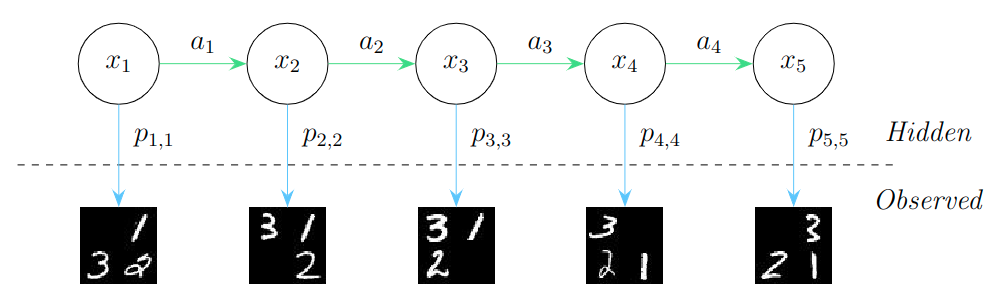### Viterbi’s Algorithm

In particular, we mention Viterbi’s algorithm. Below is a gif showing the prediction paths that are maintained throughout the algorithm, which I will expand on soon. (For now, just testing my gif!)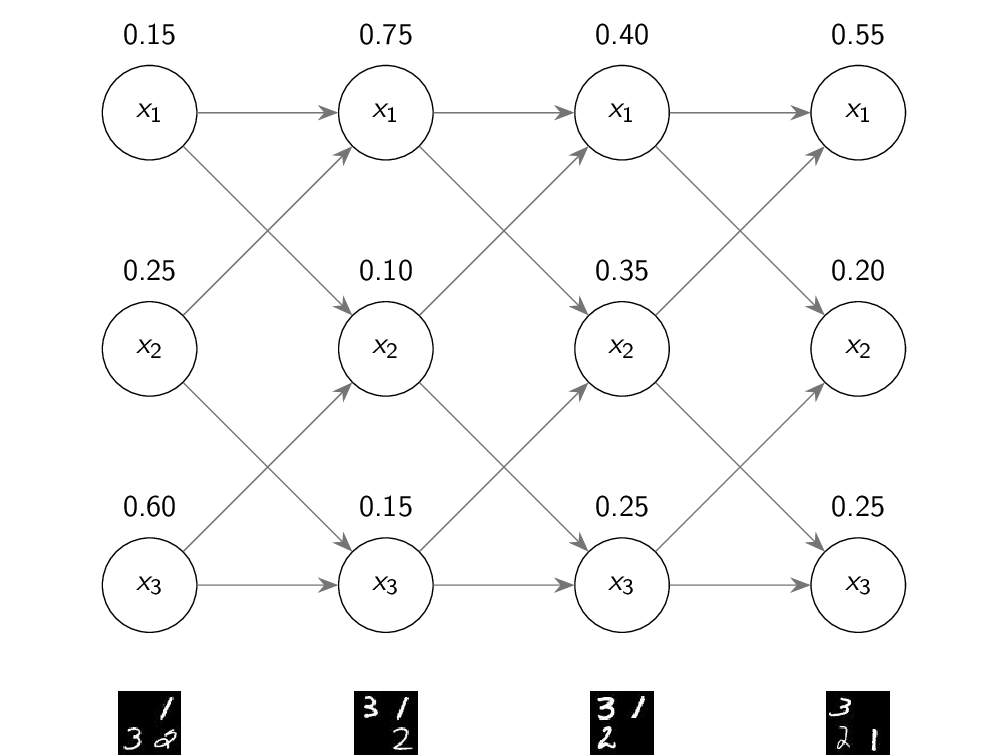I am currently in the process of updating this page as the research is still in progress. Some initial research and results can be seen in this poster I recently presented at Vector’s Research Symposium.